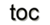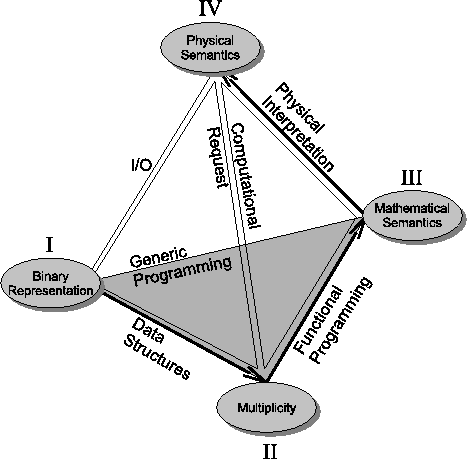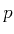Next: 4.2 Evolution of Programming Up: 4. Programming Concepts Previous: 4. Programming Concepts

# 4.1 Data Model for Scientific Computing

For the practical realization of the transition of the given theoretical concepts into applicable concepts, an effective data model is essential to the design and development of scientific software . Data exchange and data access, whether it may be internally within an application via independent software components or externally among applications, requires defined and consistent interfaces which explicitly communicate all the properties of a given dataset. Especially by the current worldwide development of shared and open-source resources, the concept of data and even application interoperability is a growing demand. The concept of a data model, denoting a data structure with a set of operations on it, is exactly this type of concept and was first considered by F. Codd   by defining a relational scheme for structuring data and providing access and query operations for relational databases. So a data model is a primary consideration in the design of any software system dealing with different data occurring in scientific computing.

Four different concepts for application design are therefore identified which are required to describe scientific data. The different requirements for the interfaces between these levels are analyzed according to their relationships, as depicted in Figure 4.1.The affinity to various programming paradigms can be identified by the respective couplings among each of these abstraction layers, where each level intrinsically requires a special transformation into software components. Therefore each link between these levels is classified by computational issues of scientific computing. The details of each level of abstraction are reviewed next:

I.)
The level of binary representation is the bottom most level of each computational design. An important fact is that high-level applications must not depend on assumptions made within this level, e.g., floating point numbers and their byte representation. For a modern application design approach and the correlated rapid development in computer hardware and computer systems, it is not even allowed for high performance calculations to depend on this layer.

II.)
The semantic topological information of multiplicity must state how many atomic types are required to describe the domain of interest, e.g., how many floating point values are required to numerically describe the coordinates of a given domain.

III.)
Mathematical semantics are related to the identification of the mathematical purpose of a certain object, e.g., the separation of a tangent vector, a normal vector, or a coordinate location, which are all represented numerically by the same number of atomic types. The mathematical properties for the given objects are defined by their chart transition rules.

IV.)
The final level of physical semantics represents the physical interpretation of a certain data set, which has to be associated with data in order to determine its purpose, e.g., given a vector field and its corresponding role in a differential equation.

While mathematical semantics (Level III) specify which operations are possible on a certain field, the specification of which operations are meaningful (Level IV) are mostly user-driven and on the application level. When the given levels of abstraction are used, the requirements for a data model are deployed. One such possible data model was therefore suggested by Butler and Pendley [20,21], who identified an abstraction for an object-oriented scientific data model of considerable generality based on the mathematical concept of fiber bundles (see Section 1.5). Its adoption was, until now, restricted to scientific visualization. Scientific visualization deals with results from large-scale simulations that generate a large amount of numerical data. The analysis of data has become an increasingly important research task, and various overviews and related implementations are available [78,80,81,1,82].

Butler's approach was originally limited to vector bundles and implemented in Eiffel . It was the first step towards a data model for scientific computing, but not without practical issues . In this approach there was no concept of cell and complex topology (cells and skeletons) on a discretized manifold. The use of different types of-cells is a fundamental requirement for arbitrary-cochains, e.g., the use of cells instead of vertices is important when interpolating data at arbitrary locations.Next: 4.2 Evolution of Programming Up: 4. Programming Concepts Previous: 4. Programming Concepts

R. Heinzl: Concepts for Scientific Computing